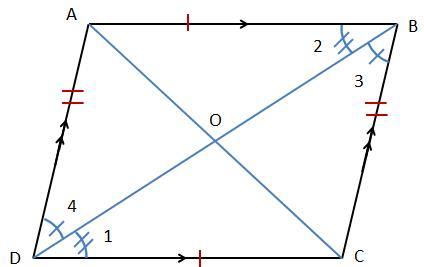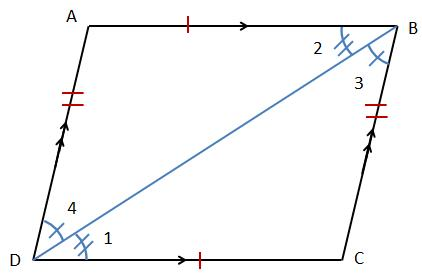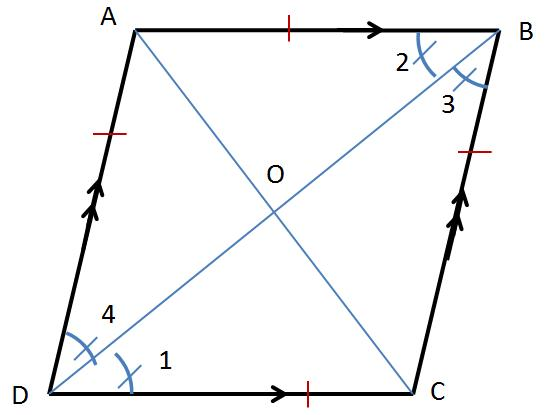Parallelogram

Definition: Parallelogram is a quadrilateral where both pairs of the opposite sides are parallel to each other.

In the figure shown,$AB\parallel DC \ and AD\parallel BC$

Note that AC is a transversal to$AB \ and\ DC$. Similarly, AC is also a transversal to$AB \ and\ DC$.

Properties of a Parallelogram (please refer to the above diagram):

1) Opposite sides are equal and parallel to each other.$AB\parallel DC \ and \ AB = DC$$AD\parallel BC \ and \ AD = BC$

2) Opposite angles are equal$\angle A =\angle C \ and\ \angle D =\angle B$

3) Adjacent angles are supplementary (consecutive interior angles)$\angle A+\angle D=180^{\circ}$$\angle C +\angle B = 180^{\circ}$$\angle C +\angle D = 180^{\circ}$$\angle A +\angle B = 180^{\circ}$

4) The diagonals (AC and BD) bisect each other$AO = OC$$DO = OB$

5) Each diagonal bisect the parallelogram in two congruent triangles

AC bisects the parallelogram ABCD in$\Delta ACD\ and\ \Delta ACB$ such that in$\Delta ACD \cong \Delta ACB$

BD bisects the parallelogram ABCD in$\Delta ABD\ and\ \Delta BCD$ such that in$\Delta ABD \cong \Delta BCD$

Theorem: In a parallelogram,Opposite sides are equal

Opposite angles are equal

Each diagonal bisects the parallelogram

Proof:

Given: Parallelogram$ABCD$ such that$AB \parallel DC\ and\ AD \parallel BC$

To Prove:$AB = DC \ and\ AD = BC$$\angle A =\angle C \ and\ \angle D =\angle B$$\Delta ABD \cong \Delta BCD$ i.e. area of$\Delta ABD$ is equal to area of$\Delta BCD$

Similarly,$\Delta ACD \cong \Delta ACB$ i.e. area of$\Delta ACD$ is equal to area of$\Delta ACB$

Proof:$\angle 1 =\angle 2$ (alternate angles because$AB \parallel DC \ and\ DB$ is a transversal)$\angle 4 =\angle 3$ (alternate angles because$AD \parallel BC \ and\ DB$ is a transversal)

DB is common

Therefore,$\Delta ABD \cong \Delta BCD$    [A.S.A axiom]

i) Since$\Delta ABD \cong \Delta BCD$$AB = DC \ and\ AD = BC$

ii) Since$\Delta ACD \cong \Delta ACB$$\angle C = \angle A$$\angle D = \angle B (since \angle 1 = \angle 2 \ and\ \angle 4 = \angle 3)$

Hence,$\angle A=\angle C \ and\ \angle D=\angle B$

Since$\Delta ABD \cong \Delta BCD$

Area of$\Delta ABD = \ Area \ of \Delta BCD$   (since congruent triangles are equal in area)

Similarly, if we were to join AC, we could use the same logic to prove$\Delta ACD \cong \Delta ACB$.

RhombusDefinition: A parallelogram where all four sides are equal is called a Rhombus.$AB \parallel DC \ and\ AD \parallel BC$$AB = DC = AD = BC$

Properties of a Rhombus:

1) Opposite sides are parallel to each other.$AB \parallel DC$$AD \parallel BC$

2) All sides are equal$AB = DC = AD = BC$

3) Diagonals AC and BD bisect each other at right angles$\angle AOB = \angle AOD = \angle BOC = \angle COD = 90^{\circ}$

4) Diagonal$BD \ bisect\ \angle A$  (i.e.$\angle ADO = \angle ODC$). Similarly, diagonal$AC \ bisects\ \angle A \ and \ \angle C.$

Similarly,$diagonal\ AC\ bisects\ \angle A \ and\ \angle C$.

Theorem: The diagonals of a Rhombus bisect each other at right angles.

Given: Rhombus$ABCD, AC \ and\ BD$ are diagonals.

To Prove:$\angle BOC=\angle COD=90^{\circ}$

Proof:$\angle ODC =\angle OBA$    (alternate angles)$\angle OCD =\angle OAB$  (alternate angles)$AB = DC$    (Side of a Rhombus)

Therefore$\Delta AOB \cong \Delta COD$   (A.S.A Axiom)

Hence$OD = OB \ and\ OA = OC$

Again in$\Delta ODC\ and\ \Delta OBC$$DC = BC$  (Side of a Rhombus)$OD = OB$ (Proved above) And$OC$  is common

Hence$\Delta ODB \cong \Delta OBC$   (S.S.S axiom)

Therefore$\angle DOC =\angle COB$

Now$\angle DOC +\angle COB =180^{\circ}$

Or$\angle DOC =\angle COB =90^{\circ}$